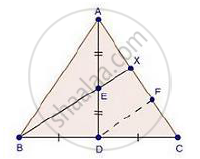Share
Notifications

View all notifications
Books Shortlist
Your shortlist is empty

# Solution for D is the Mid-point of Side Bc of a δAbc. Ad is Bisected at the Point E and Be Produced Cuts Ac at the Point X. Prove that Be : Ex = 3 : 1 - CBSE Class 10 - Mathematics

Login
Create free account

Forgot password?
ConceptCriteria for Similarity of Triangles

#### Question

D is the mid-point of side BC of a ΔABC. AD is bisected at the point E and BE produced cuts AC at the point X. Prove that BE : EX = 3 : 1

#### SolutionGiven: In ΔABC, D is the mid-point of BC and E is the mid-point of AD.

To prove: BE : EX = 3 : 1

Const: Through D, draw DF || BX

Proof: In ΔEAX and ΔADF

∠EAX = ∠ADF                         [Common]

∠AXE = ∠DAF                         [Corresponding angles]

Then, ΔAEX ~ ΔADF                [By AA similarity]

therefore"EX"/"DF"="AE"/"AD"                      [Corresponding parts of similar Δ are proportional]

rArr"EX"/"DF"="AE"/"2AE"           [AE = ED given]

⇒ DF = 2EX …. (i)

In ΔCDF and ΔCBX               [By AA similarity]

therefore"CD"/"CB"="DF"/"BX"                [Corresponding parts of similar Δ are proportional]

rArr1/2="DF"/"BE + EX"         [BD = DC given]

⇒ BE + EX = 2DF

⇒ BE + EX = 4EX

⇒ BE = 4EX – EX                         [By using (i)]

⇒ BE = 4EX – EX

rArr"BE"/"EX"=3/1

Is there an error in this question or solution?

#### Video TutorialsVIEW ALL 

Solution D is the Mid-point of Side Bc of a δAbc. Ad is Bisected at the Point E and Be Produced Cuts Ac at the Point X. Prove that Be : Ex = 3 : 1 Concept: Criteria for Similarity of Triangles.
S